Ch 4. The Mean Value Theorem Multimedia Engineering Math Maximum & Minimum Rolle'sTheorem Mean ValueTheorem MonotonicFunctions First DerivativeTest Concavity &Inflection SecondDerivative Test
 Chapter 1. Limits 2. Derivatives I 3. Derivatives II 4. Mean Value 5. Curve Sketching 6. Integrals 7. Inverse Functions 8. Integration Tech. 9. Integrate App. 10. Parametric Eqs. 11. Polar Coord. 12. Series Appendix Basic Math Units Search eBooks Dynamics Fluids Math Mechanics Statics Thermodynamics Author(s): Hengzhong Wen Chean Chin Ngo Meirong Huang Kurt Gramoll ©Kurt GramollMATHEMATICS - THEORY Mean Value Theorem and its geometry meaning are discussed in this section. Mean Value Theorem In the previous section, Rolle's theorem was introduced. One of the prerequisite of that theorem is the two endpoints value are equal. However, the mean value theorem does not have this requirement. It is more general than the Rolle's theorem. The mean value theorem states that if a function satisfies the following two hypotheses, the function is continuous on the closed interval [a, b], the function is differentiable on the open interval (a, b), then there is a point c in the open interval (a, b) such that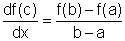(1)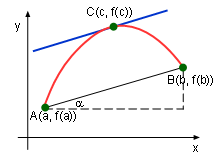Mean Value Theorem Explanation Graphic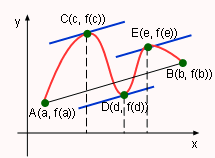Multiple Parallel Line Rearranging this equation gives: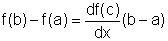(2) Now consider the slope of the line AB,      mAB = tanα = (f(b)-f(a))/(b-a)                          (3) The right hand side of the equation (1) and (3) are equal. Since the left hand side of equation (1) is the slope of the tangent line at point C, the mean value theorem means that there is a pont C where the tangent line is parallel to the line AB. If a function satisfies the hypotheses of the mean value theorem, it may have multiple function tangent lines that are parallel to the secant line AB. The mean value theorem is important because it sets some basic facts in differential calculus. One of these basic facts is the following theorem. Theorem Related to Constant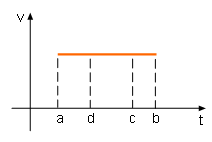Velocity is Constant One of the example in Rolle's Theorem is a car driving at an uniform speed, its acceleration is 0. It can also be stated that if a car has zero acceleration, then its velocity is uniform. This is true in the physics, and it is an example of how the theorem works. The theorem states: If the derivative of a function at all points are 0 in an open interval (a, b), then the function is constant on (a, b). Is there any case that this theorem fails? Consider another example,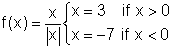The function is obviously not a constant. Does theorem fail in this function? The answer is no. Since when x equals 0, this function is not differentiable. In (0, +∞) and ( -∞, 0) the function are constant.